# Example Question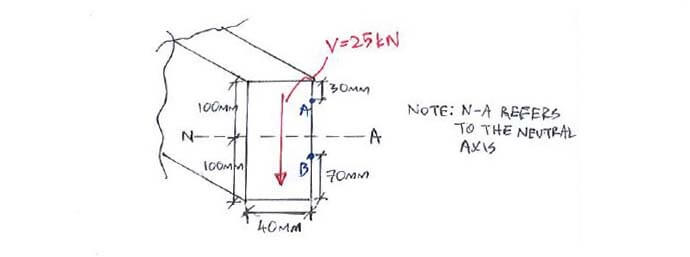Click below to show answer. X

#### Step 1: Calculate moment of inertia I Step 1: Calculate moment of inertia I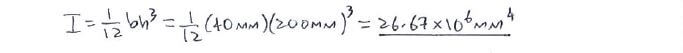#### Step 2: Calculate Q at A, B and neutral-axis Step 2: Calculate Q at A, B and neutral-axis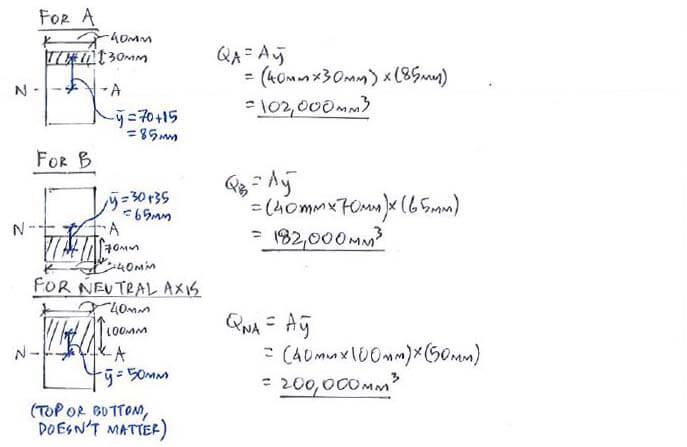#### Step 3: Calculate transverse shear stress Step 3: Calculate transverse shear stress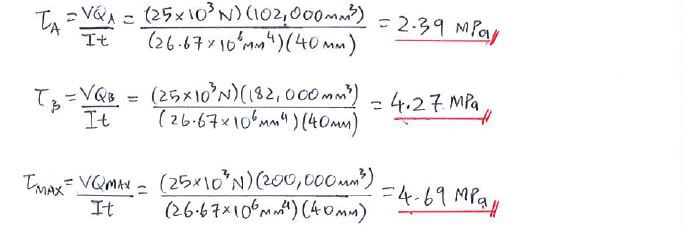#### Useful formula: Useful formula: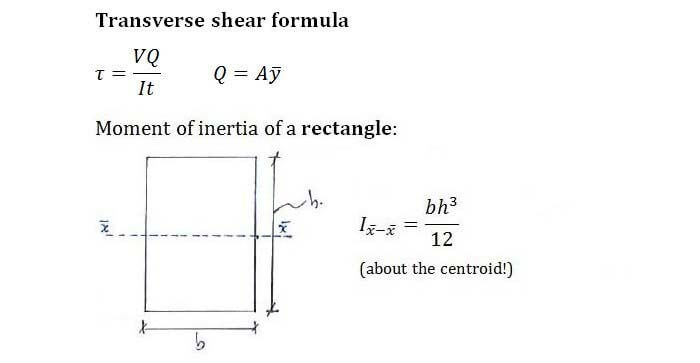Now that you've mastered this question, you can try Question 1.